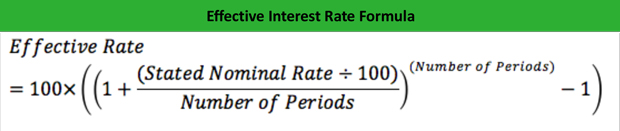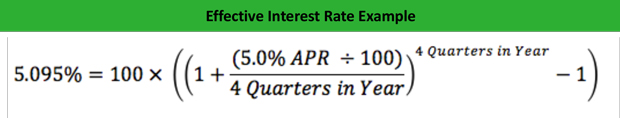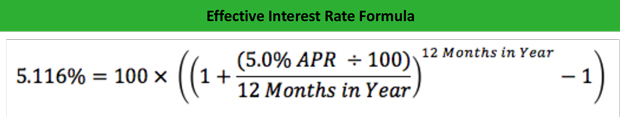# What is the Effective Annual Interest Rate?

Definition: The effective annual interest rate, or annual equivalent rate, earned or paid is the actual rate realized when taking into account compounding interest periods.

## What Does Effective Interest Rate Mean?

What is the definition of effective interest rate? Based on the stated or nominal rate for a given period, such as an annual interest rate, the effective rate is calculated by incorporating the impact of compounding interest periods into the stated, nominal rate. The effective interest rate formula is calculated like this:The purpose of calculating the effective rate on any financial instrument is to gain an accurate understanding of the true interest earned or paid over a period of time. While a financial institution may offer something like a mortgage loan with an annual percentage rate of 4.5%, it’s critical to understand that the number of periods the interest is paid during the annual term will impact the true effective rate of the loan.

Let’s dive into a basic example.

## Example

Let’s pretend it’s your lucky day, and you win a lump sum prize of cash through a local drawing. After receiving your lump sum payout, and paying the necessary taxes and fees, you walk away with \$10,000! Now assuming you don’t depend on the money, you decide you’re going to deposit the money into a safe investment, like a Certificate of Deposit at a local bank. Two banks in your area have attractive offers, Bank A offers a 5 year Certificate of Deposit with an annual percentage rate of 4%, compounding quarterly, and Bank B offers a 5 year Certificate of Deposit with an annual percentage rate of 4%, compounding monthly.

Simply put, the rules of compounding interest state that the more times interest compounds in a given period, the better the effective rate, but for purposes of our example, we want to understand the true effective rate of each option, and how much it will make us!

Leveraging the equation above, Bank A would be offering an effective interest rate of 5.095% per year, calculated with the following equation:Bank B would be offering and effective rate of 5.116% per year, calculated with the following equation:As a result of quick calculation, it would be in your best interest to deposit your winnings into Bank B’s Certificate of Deposit offering!

## Summary Definition

Define Effective Interest Rate: Effective annual rate means the actual interest from an instrument calculated using compound interest.